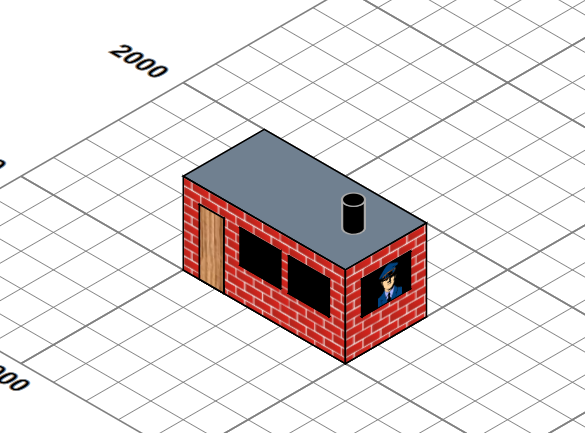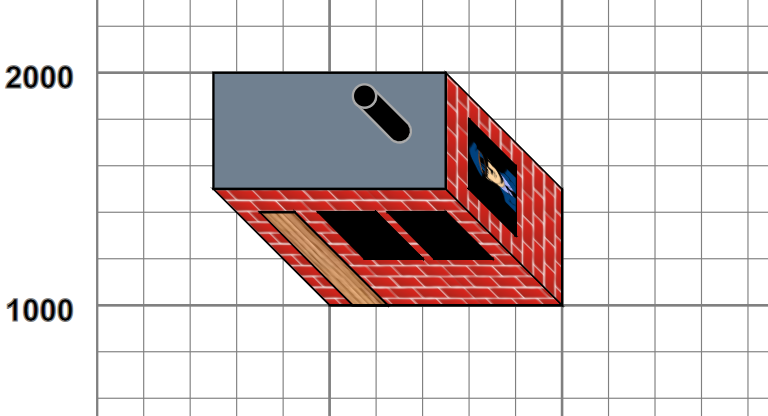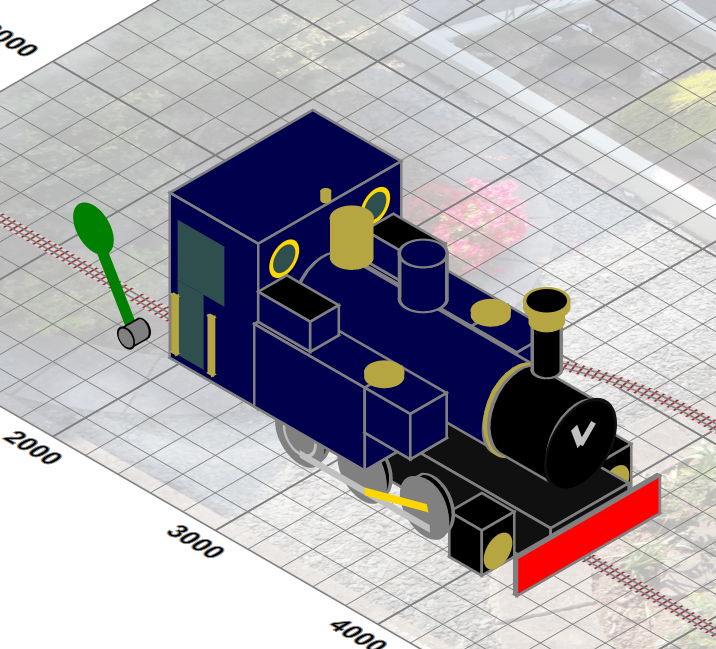### Isometric Views

Planar views are useful, but they don't give a picture of what one might see, where the third dimension has some importance. Luckily an isometric transformation can give a view from above and aside. This involves applying a transform of `translate(3000,0) rotate(30) skewX(-30) scale(1,0.8660254037844387)` to the graphics and altering some pieces to support a pseudo-3D view. For example, let us add a simple building:

Figure 11. A simple buildingI could perhaps have looked at using a full 3D modelling package which was capable of generating SVG outputs, but my needs were modest and could perhaps be handled by a simple declarative model, processed completely with XSLT to generate suitable SVG. The building is defined by a simple XML structure of boxes and a cylinder:

```<buildings>
<resources> …. </resources>
<group x="1000" y="1000"
fill="url(#brickWall)" stroke-width="10" stroke="black">
<box width="1000" height="500" rotateZ="0" depth="500" z="0">
<top fill="slategrey"/>
</box>
<box height="1" width="150" depth="400"
z="0" x="100" y="0" fill="url(#wood)"/>
<box height="1" width="250" depth="200" fill="black"
z="200" x="350" y="0" />
<box height="1" width="250" depth="200" fill="black"
z="200" x="650" y="0"/>
<box width="1" height="300" depth="200" fill="black"
z="200" x="1000" y="100">
<east >
x="100" y="0" height="200"/>
</east>
</box>
z="500" x="800" y="250" stroke="darkgrey"/>
</group>
</buildings>```

which is then used to generate an SVG group that look like:

Figure 12. An iso-orthogonal buildingsuch that when the entire SVG group, within which lie all picture pieces (grid, plan, track etc..), is subject to isometric projection, the building appears to have depth and height. (We also produce a true orthogonal view, so we can look at the scene from directly above.) Currently the repertoire is orthogonally-oriented rectangular blocks and cylinders, with named faces to which styling and content can be attached (top, south and east for blocks, with bottom, north and west normally hidden, and surface, top and bottom for cylinders.). Components are currently positioned absolutely and can be grouped. Using this we can build models of the complexity of:which is defined by some 50 components, some of which are repeats of common substructures, implemented by bindings and interpolations of XSLT variables. This ability to style and add content to the named faces of the component parts is important . For example, adding the smokebox handle to the boiler front of Lady Anne merely requires:

```<cylinder class="boilerFront" x="151" z="80" axis="x"